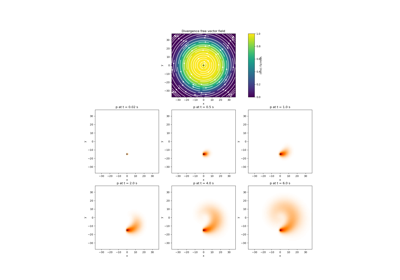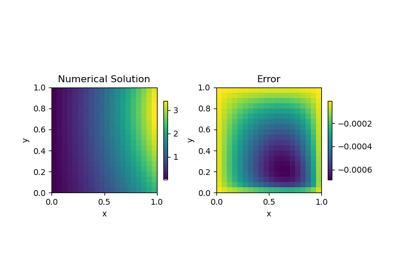# discretize.base.BaseMesh#class discretize.base.BaseMesh[source]#

Base mesh class for the discretize package.

This class contains the basic structure of properties and methods that should be supported on all discretize meshes.

Attributes

 area area has been deprecated. average_cell_to_edge Averaging operator from cell centers to edges (scalar quantities). average_cell_to_face Averaging operator from cell centers to faces (scalar quantities). average_cell_vector_to_face Averaging operator from cell centers to faces (vector quantities). average_edge_to_cell Averaging operator from edges to cell centers (scalar quantities). average_edge_to_cell_vector Averaging operator from edges to cell centers (vector quantities). average_edge_to_face Averaging operator from edges to faces. average_face_to_cell Averaging operator from faces to cell centers (scalar quantities). average_face_to_cell_vector Averaging operator from faces to cell centers (vector quantities). average_node_to_cell Averaging operator from nodes to cell centers (scalar quantities). average_node_to_edge Averaging operator from nodes to edges (scalar quantities). average_node_to_face Averaging operator from nodes to faces (scalar quantities). boundary_edge_vector_integral Represent the operation of integrating a vector function on the boundary. boundary_edges Boundary edge locations. boundary_face_outward_normals Outward normal vectors of boundary faces. boundary_face_scalar_integral Represent the operation of integrating a scalar function on the boundary. boundary_faces Boundary face locations. boundary_node_vector_integral Represent the operation of integrating a vector function dotted with the boundary normal. boundary_nodes Boundary node locations. cell_centers Return gridded cell center locations. cell_volumes Return cell volumes. dim The dimension of the mesh (1, 2, or 3). edge edge has been deprecated. edgeCurl edgeCurl has been deprecated. edge_curl Edge curl operator (edges to faces). edge_lengths Return lengths of all edges in the mesh. edge_tangents Unit tangent vectors for all mesh edges. edges Gridded edge locations. faceDiv faceDiv has been deprecated. face_areas Return areas of all faces in the mesh. face_divergence Face divergence operator (faces to cell-centres). face_normals Unit normal vectors for all mesh faces. faces Gridded face locations. n_cells Total number of cells in the mesh. n_edges Total number of edges in the mesh. n_faces Total number of faces in the mesh. n_nodes Total number of nodes in the mesh. nodalGrad nodalGrad has been deprecated. nodalLaplacian nodalLaplacian has been deprecated. nodal_gradient Nodal gradient operator (nodes to edges). nodal_laplacian Nodal scalar Laplacian operator (nodes to nodes). nodes Return gridded node locations. normals normals has been deprecated. project_edge_to_boundary_edge Projection matrix from all edges to boundary edges. project_face_to_boundary_face Projection matrix from all faces to boundary faces. project_node_to_boundary_node Projection matrix from all nodes to boundary nodes. stencil_cell_gradient Stencil for cell gradient operator (cell centers to faces). tangents tangents has been deprecated. vol vol has been deprecated.

Methods

 closest_points_index(locations[, grid_loc, ...]) Find the indicies for the nearest grid location for a set of points. Make a copy of the current mesh. deserialize(items, **kwargs) Create this mesh from a dictionary of attributes. equals(other_mesh) Compare the current mesh with another mesh to determine if they are identical. getEdgeInnerProduct(*args, **kwargs) getEdgeInnerProduct has been removed. getEdgeInnerProductDeriv(*args, **kwargs) getEdgeInnerProductDeriv has been removed. getFaceInnerProduct(*args, **kwargs) getFaceInnerProduct has been removed. getFaceInnerProductDeriv(*args, **kwargs) getFaceInnerProductDeriv has been removed. getInterpolationMat(*args, **kwargs) getInterpolationMat has been removed. get_edge_inner_product([model, ...]) Generate the edge inner product matrix or its inverse. get_edge_inner_product_deriv(model[, ...]) Get a function handle to multiply vector with derivative of edge inner product matrix (or its inverse). get_face_inner_product([model, ...]) Generate the face inner product matrix or its inverse. get_face_inner_product_deriv(model[, ...]) Get a function handle to multiply a vector with derivative of face inner product matrix (or its inverse). get_interpolation_matrix(loc[, ...]) Construct a linear interpolation matrix from mesh. point2index(locs) Find cells that contain the given points. projectEdgeVector(*args, **kwargs) projectEdgeVector has been removed. projectFaceVector(*args, **kwargs) projectFaceVector has been removed. project_edge_vector(edge_vectors) Project vectors to the edges of the mesh. project_face_vector(face_vectors) Project vectors onto the faces of the mesh. save([file_name, verbose]) Save the mesh to json. Represent the mesh's attributes as a dictionary. Represent the mesh's attributes as a dictionary. Return the validation state of the mesh.

## Galleries and Tutorials using discretize.base.BaseMesh#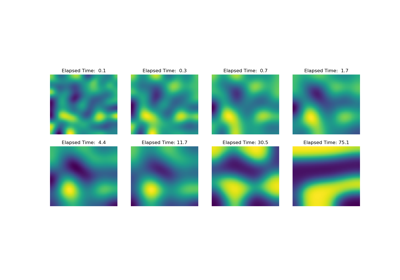Operators: Cahn Hilliard

Operators: Cahn Hilliard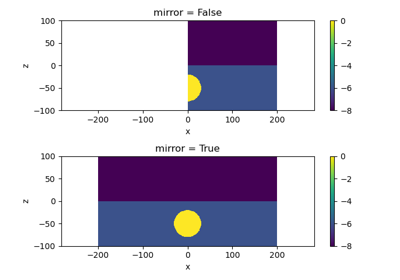Plot Mirrored Cylindrically Symmetric Model

Plot Mirrored Cylindrically Symmetric Model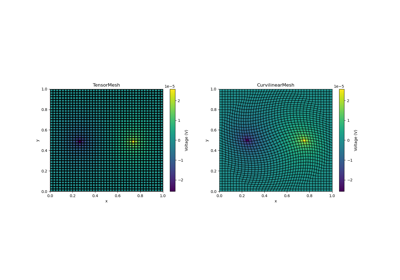Basic Forward 2D DC Resistivity

Basic Forward 2D DC ResistivityBasic: PlotImage

Basic: PlotImage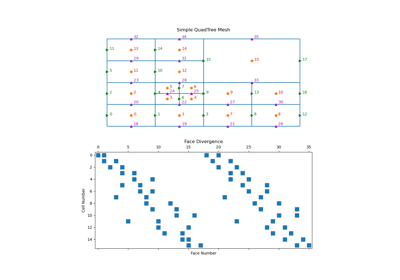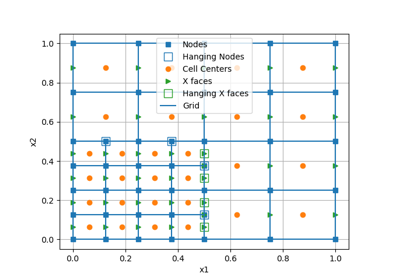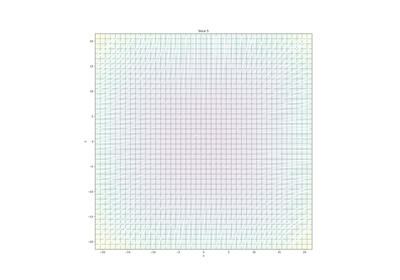Plotting: Streamline thickness

Plotting: Streamline thickness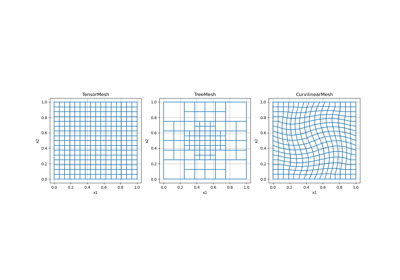Overview of Mesh Types

Overview of Mesh Types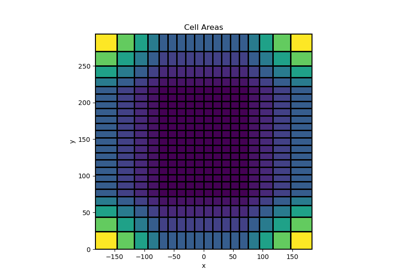Tensor meshes

Tensor meshes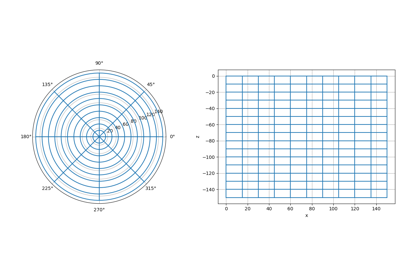Cylindrical meshes

Cylindrical meshes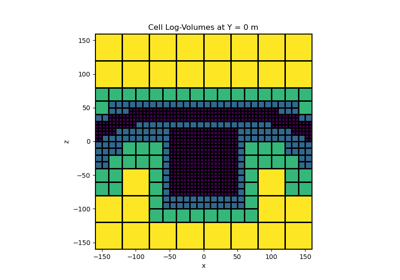Tree Meshes

Tree Meshes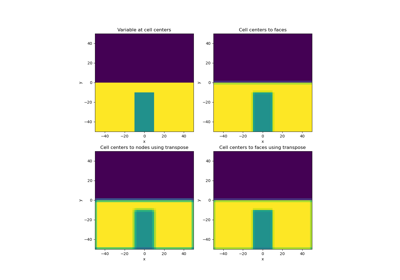Averaging Matricies

Averaging Matricies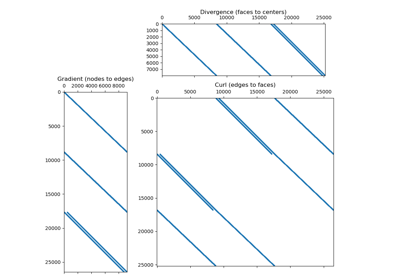Differential Operators

Differential Operators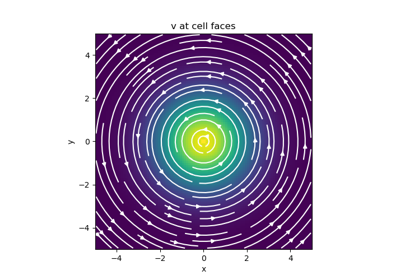Basic Inner Products

Basic Inner Products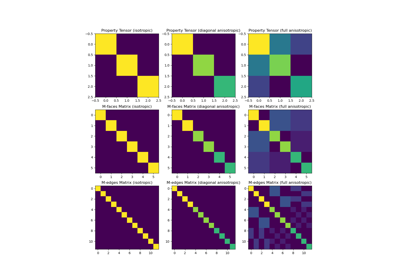Constitutive Relations

Constitutive Relations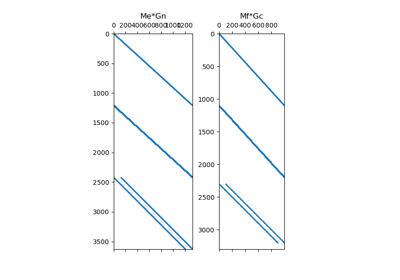Differential Operators

Differential Operators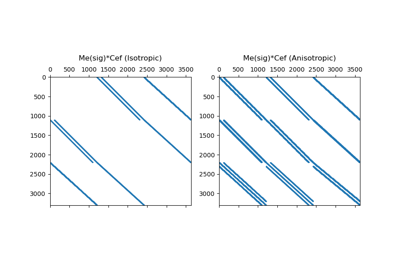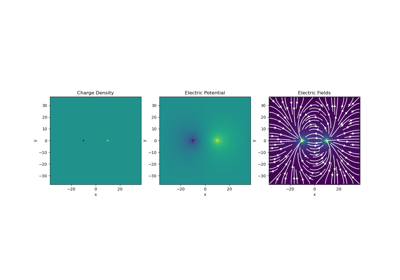Gauss’ Law of Electrostatics

Gauss' Law of Electrostatics